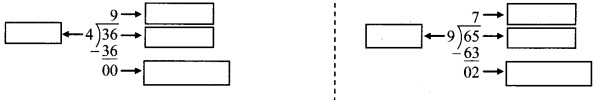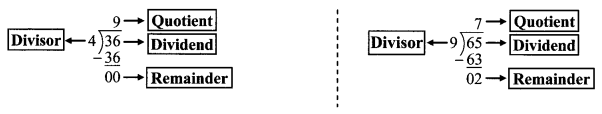# Maharashtra Board Practice Set 23 Class 6 Maths Solutions Chapter 9 HCF-LCM

Balbharti Maharashtra State Board Class 6 Maths Solutions covers the Std 6 Maths Chapter 9 HCF-LCM Class 6 Practice Set 23 Answers Solutions.

## 6th Standard Maths Practice Set 23 Answers Chapter 9 HCF-LCM

Question 1.
Write all the factors of the given numbers and list their common factors:
i. 12, 16
ii. 21, 24
iii. 25, 30
iv. 24, 25
v. 56, 72
Solution:
i. Factors of 12 = 1, 2, 3, 4, 6, 12
Factors of 16 = 1, 2, 4, 8, 16
∴ Common factors of 12 and 16 = 1, 2, 4

ii. Factors of 21 = 1, 3, 7, 21
Factors of 24 = 1, 2, 3, 4, 6, 8, 12, 24
∴ Common factors of 21 and 24 = 1, 3

iii. Factors of 25 = 1, 5, 25
Factors of 30 = 1, 2, 3, 5, 6, 10, 15, 30
∴ Common factors of 25 and 30 = 1, 5

iv. Factors of 24 = 1, 2, 3, 4, 6, 8, 12, 24
Factors of 25 = 1,5, 25
∴ Common factor of 24 and 25 = 1

v. Factors of 56 = 1, 2, 4, 7, 8, 14, 28, 56
Factors of 72 = 1, 2, 3, 4, 6, 8, 9, 12, 18, 24, 36, 72
∴ Common factors of 56 and 72 = 1, 2, 4, 8

#### Maharashtra Board Class 6 Maths Chapter 9 HCF-LCM Practice Set 23 Intext Questions and Activities

Question 1.
In the empty boxes, write the proper words: dividend, divisor, quotient, remainder. (Textbook pg. no. 46)Solution:When we divide 36 by 4, the remainder is zero. Therefore, 4 is a factor of 36 and 36 is a multiple of 4. But, when we divide 65 by 9, the remainder is not zero. Therefore, 9 is not a factor of 65. Also, 65 is not a multiple of 9.

Question 2.
Write all the factors of the numbers 36 and 48. Also, list their common factors. (Textbook pg. no. 46)
Solution:
36 = 1 × 36
= 2 × 18
= 3 × 12
= 4 × 9
= 6 × 6

48 = 1 × 48
= 2 × 24
= 3 × 16
= 4 × 12
= 6 × 8

∴ Factors of 36: 1, 2, 3, 4, 6, 9, 12, 18, 36
Factors of 48: 1, 2, 3, 4, 6, 8, 12, 16, 24, 48
Common factors of 36 and 48:  ,, , , , 

Scroll to Top